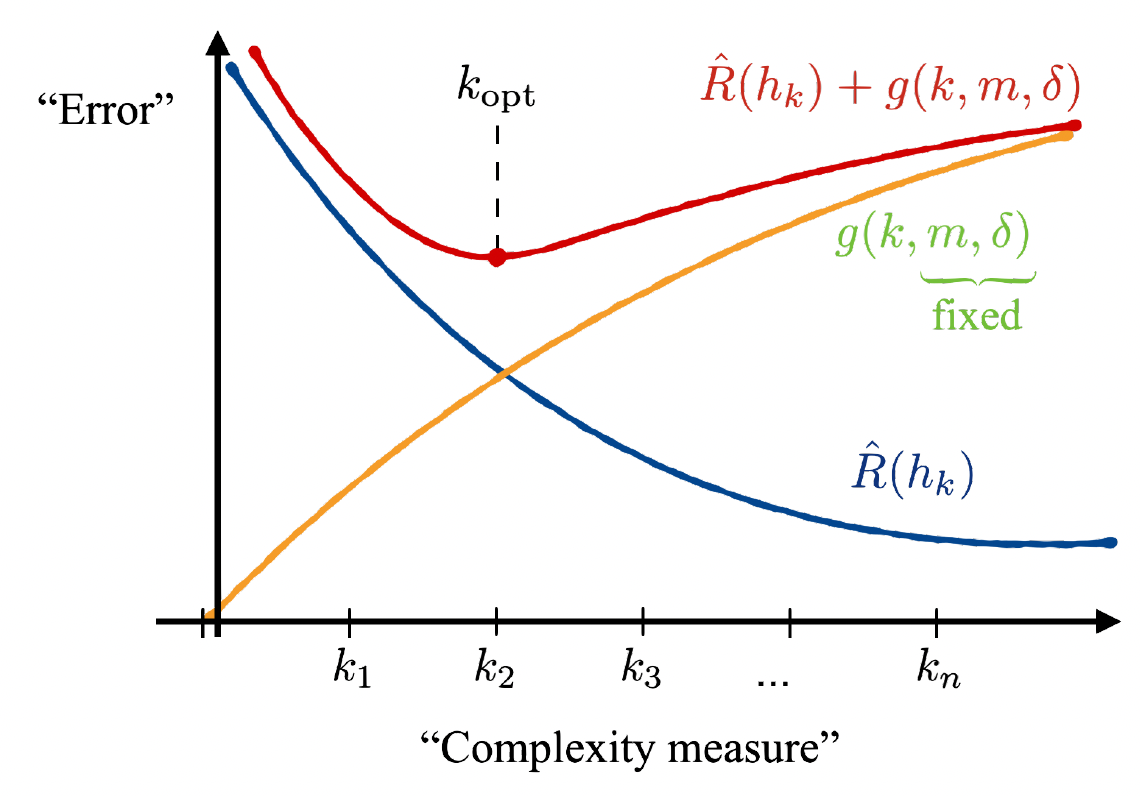Springe direkt zu Inhalt

# Work on quantum machine learning in QuantumGeneralization bounds in quantum-assisted machine learning.

How well do quantum-assisted machine learning algorithms perform on unseen data? New work pushlished in Quantum is addressing this question. A large body of recent work has begun to explore the potential of parametrized quantum circuits (PQCs) as machine learning models, within the framework of hybrid quantum-classical optimization. In particular, theoretical guarantees on the out-of-sample performance of such models, in terms of generalization bounds, have emerged. However, none of these generalization bounds depend explicitly on how the classical input data is encoded into the PQC. We derive generalization bounds for PQC-based models that depend explicitly on the strategy used for data-encoding. These imply bounds on the performance of trained PQC-based models on unseen data. Moreover, our results facilitate the selection of optimal data-encoding strategies via structural risk minimization, a mathematically rigorous framework for model selection. We obtain our generalization bounds by bounding the complexity of PQC-based models as measured by the Rademacher complexity and the metric entropy, two complexity measures from statistical learning theory. To achieve this, we rely on a representation of PQC-based models via trigonometric functions. Our generalization bounds emphasize the importance of well-considered data-encoding strategies for PQC-based models.

News from Nov 01, 2021

10 / 100